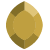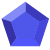# Negative Euler Deconvolution solutionsPosts: 32Hello everyone
I am a Geosoft beginner
Please I wish to know what is the meaning of negative depth solutions in Euler. Are they to dismiss from the solution list ???

•Posts: 94We ran a webinar recently on the Science Behind Euler Deconvolution which you might find useful. It focused on the application of Euler to calculate depths for unexploded ordnance but has a great section by Dr. Alan Reid on the theory of how Euler works.

http://www.geosoft.com/videos/science-behind-euler-deconvolution-how-it-affects-uxo-depth-and
Lorraine Godwin•Posts: 146edited June 2018
Hello @QUENTINMARCANABAFOTZ,

Negative depth solutions in Euler Deconvolution can occur if you use the "Survey Elevation" parameter, which can entered by the user to account for the elevation of the sensor (magnetometer/gravity meter) when calculating the Euler solutions. To clarify, the Survey Elevation is defined as the elevation above the ground surface

For example, let's assume that a user sets the Survey Elevation parameter to zero and then calculates a single Euler solution, one which is calculated as being 1000m below the sensor elevation. In this case the results database the "Elevation" for this solution will be shown as 1000m

Let us now assume that a user enters a Survey Elevation value of 500m and then calculates a single Euler solution at the same depth and location as described in the previous paragraph. In this case, the results database will show an "Elevation" value of -500m for the solution, because the Survey Elevation is accounted for.

You should therefore not delete an Euler solution just because it is negative, if you have a survey elevation defined.

Outside of those negative solutions resulting from the Survey Elevation, we do occasionally see negative results in the Depth channel or positive results in the Elevation channel. These values suggest targets above ground and this can occur for various reasons:

-Mismatch of units: If there is a mismatch between your grid units, database units and general workspace units then this can cause strange depths to be returned. It is recommended that you check the original grids as well as the grids created in the preprocessing steps (E3PREP.GX).

-Grid cell and window size: The resolution of the grid plays a role in the accuracy and values of the Euler depths. The maximum depths returned are about twice the window size; and for deep sources it is better to increase the grid cell size rather than the window size. This should increase the speed and prevent any confusion due to many small sources within the search window.

-Structural Index: Make sure to set a correct SI for the sources (geologic model) you expect. Keep in mind that if the index is too low the depths returned are too shallow and if the index is too high then the depths are too deep. Also depth estimates are more precise for high index sources than low index sources. Depth estimates also assume only one source and therefore if there is more than one source you could get spurious results.

-Mathematical uncertainty: occasionally results will be output that fulfill the mathematical criteria, but are not geologically sound.

I personally find the Survey Elevation parameter a little confusing and restrictive, so prefer to leave it set to zero and then following Euler Deconvolution I will manually derive either a depth from surface value or an elevation for each solution.

Best of luck with your project,

Darren
Darren Andrews
Technical Team Manager•Posts: 32let's assume we set the survey elevation to 0 to calculate euler solutions, will the solutions from Euler represent depths below ground surface? if not how to derive depth from surface value?
•Posts: 146edited August 2018
@QUENTINANABAFOTZE If the survey elevation is set to zero, then the Euler solution depths will be relative to the elevation at which the data was collected. For example, typically I work with magnetic data that is flown at a nominal height of 100m above ground surface. When I run Euler deconvolution on that data, I subtract that 100m from the Euler solution depths in order to make them relative to the ground surface.
Darren Andrews
Technical Team Manager•Posts: 32•Posts: 1Hi everyone, I have a related question. What happens if I didn't specify a survey elevation (I'm working with satellite gravity data) but some of the depth solutions are negative. Are these depth values related to sea level?

Greetings,
PhD student Claudia Peredo
• Hi @ClaudiaPeredo, if you did not specify a survey elevation, it will use "flying height" which by default is set to zero.

https://my.geosoft.com/supportcentre#/help/oasismontaj-extensions-e3decon_gx.htm
Community ManagerThis discussion has been closed.